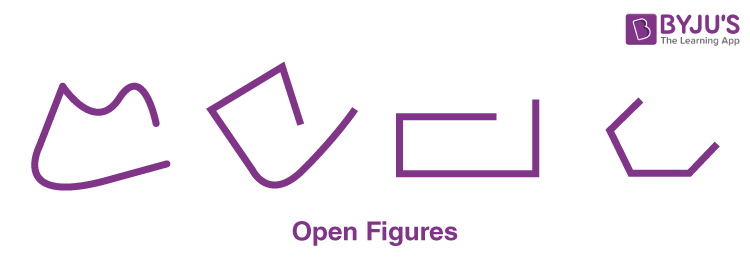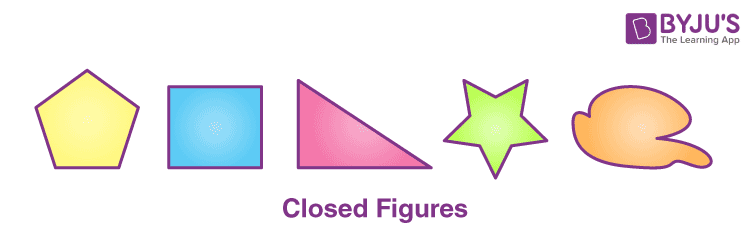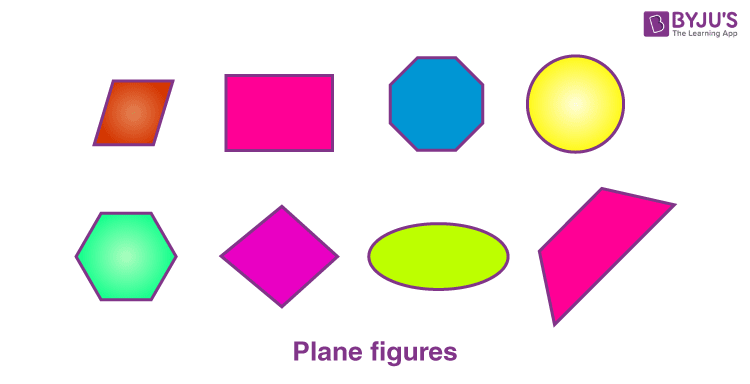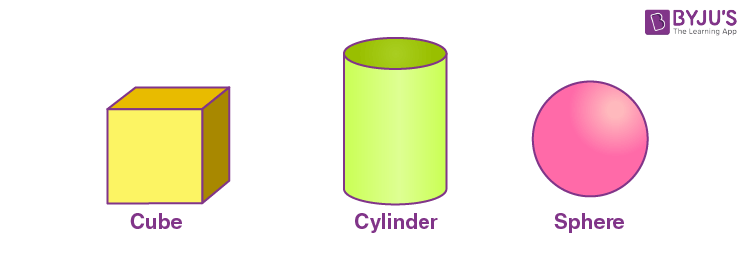# Geometrical Figures

Geometry is a branch of Mathematics used to draw and measure figures.

The word geometry refers to field measurement. We learn how to draw various figures in geometry, such as rectangles, squares, triangles, cones, cylinders and spheres, etc.

## Basic Elements of Geometry

Point

• The basic element of geometry is the point.
• A point has no length, breadth, or height.

Line• A line has only length, not breadth.
• A line segment can be straight or curved.
• A line segment can contract by joining two points.
• The shortest line segment which joins two prints is called the straight line segment.
• Figures can be drawn by joining line segments.
• Some geometrical shapes can be drawn by joining straight line segments, curved line segments, and by joining both straight and curved line segments.

### Types of Geometry Shapes

In geometry, there are two types of shapes:

Open FiguresOpen figures are open from all sides.

Closed FiguresClosed figures are closed from all sides

Parallel to the open and closed figures, some plane figures and solid shapes are discussed below.

Plane Figures

Plane figures have length and breadth and are drawn by joining line segments. Triangles, quadrilaterals, rectangles, squares, and circles are some examples of plane figures.Solid Shapes

Solid shapes have length, breadth and height. Cubes, cuboids, cylinders, spheres are some examples of solid shapes.Stay tuned to BYJU’S for more information on NIOS, syllabus, notes, along with its important questions and solutions.